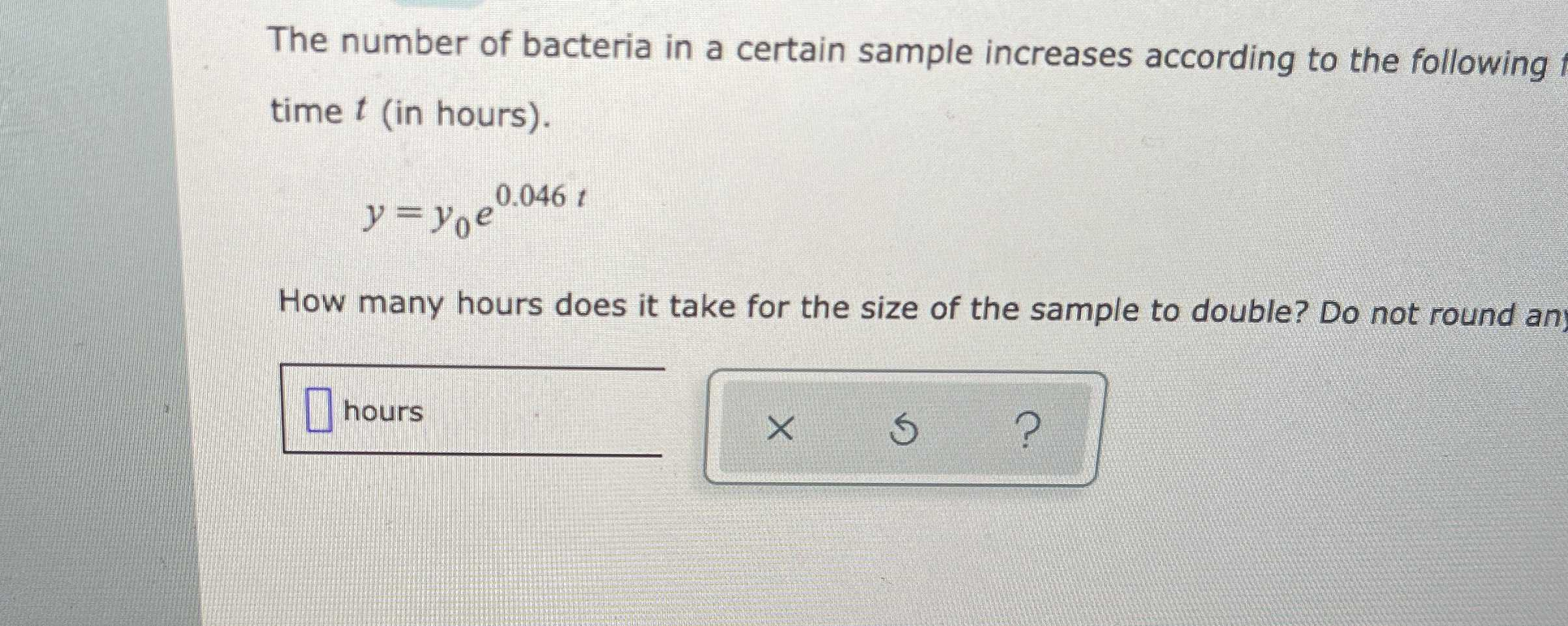### ¿Todavía tienes preguntas de matemáticas?

Pregunte a nuestros tutores expertos
Algebra
Pregunta0.046t

The number of bacteria in a certain sample increases according to the following time $$t$$ (in hours).

$$y = y _ { 0 } e ^ { 0.046 t }$$

$$e^{0.046t}= 2\\t= \frac{\ln 2}{0.046}$$